Technical Article

# Understanding Limitations to Increasing SFDR in High-speed ADCs

April 30, 2023 by Dr. Steve Arar

## Learn about two nonlinearity sources, spurious-free dynamic range (SFDR), and SNR (signal-to-noise ratio) in analog-to-digital converters (ADCs).

The spurious-free dynamic range (SFDR) is a common way to characterize the linearity performance of a circuit. This specification is particularly helpful when dealing with communications systems. Examining the general functionality of A-D converters (ADCs), this article tries to explain the two main nonlinearity sources, namely the sample and hold (S/H) circuit and the encoder portion of the ADC, that limit the ADC SFDR performance.

We’ll also learn about a general trade-off between SFDR and SNR (signal-to-noise ratio) in ADCs as well as laying the foundation for an interesting discussion in a future article about applying the dithering technique to improve ADC SFDR. Dithering is the technique of deliberately adding an appropriate noise component to the ADC input to improve certain performance aspects of the A-D conversion system. It sounds like magic thinking that adding noise can improve SFDR.

However, before diving in too far, let’s have a quick review of what SFDR is and why it is important.

### What is Spurious Free Dynamic Range (SFDR)? Why is SFDR Important?

There are several different specifications that can be used to characterize circuit linearity. One specification that is popular to use is the SFDR metric. This metric is defined as the ratio of the desired signal amplitude to the largest spur over the bandwidth of interest (Figure 1).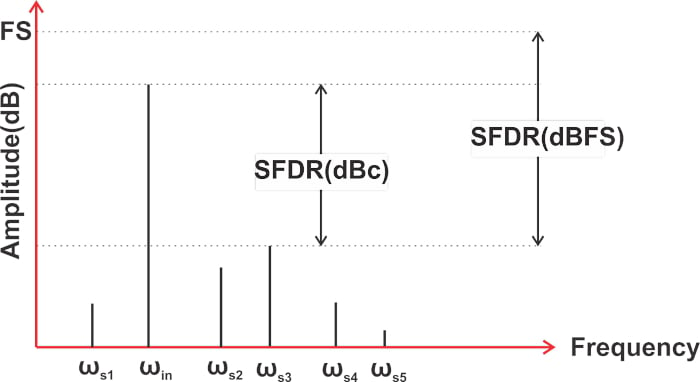##### Figure 1. Chart showing SFDR metric.

When it comes to ADCs, the SFDR shows how the ADC can simultaneously process a small signal in the presence of a large signal. As an example, consider a receiver application. Suppose that the ADC input consists of a +1 dBm blocker and a -75 dBm desired signal. In this case, the large blocker can create unwanted spurs at the ADC output because of the ADC nonlinearity. These unwanted spurs are shown by purple components in Figure 2.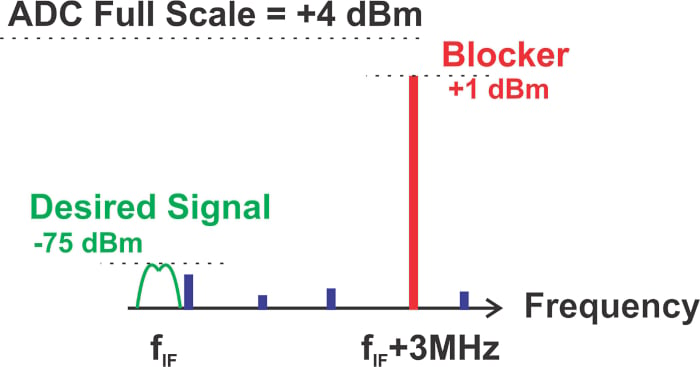##### Figure 2. A graph showing the unwanted spurs in purple.

If a spur is close enough to the desired signal and is sufficiently large, it can degrade the SNR to an unacceptable level. The rigorous demands of today’s communications systems can require high SFDR values in the range of 95 dB. However, a garden-variety ADC cannot provide this level of linearity. Below, Table 1, which compares some key parameters of four high-performance ADCs from Analog Devices, should help you have an idea about the SFDR range in high-performance ADCs.

##### Table 1. Key parameters of four high-performance ADCs. Data used courtesy of Analog Devices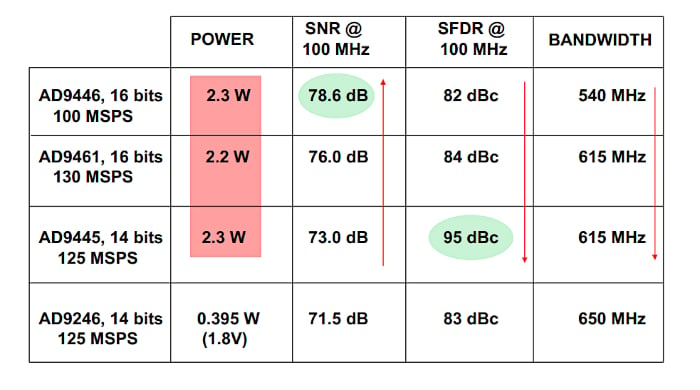Additionally, this table highlights a trade-off between the SNR and SFDR metrics. For the first three ADCs in this table, which use the same IC technology and have identical power consumption, there is an inverse relationship between the SFDR and SNR. We’ll get into the origin of this trade-off a little later in this article. Before that, let’s answer an important question: what are the major limitations to increasing SFDR in a high-speed ADC?

### Static and Dynamic Linearity in an ADC

ADCs are complex systems designed based on a number of different circuit architectures, such as flash, SAR, delta-sigma (ΔΣ), and pipeline structures. Depending on the architecture and the particular circuit implementation, different circuit components can be the major source of nonlinearity. Although there are numerous designs, we can still recognize two major limitations to increasing SFDR in a high-speed ADC, namely the S/H circuit and the encoder portion of the ADC. To better understand this, consider the block diagram of a SAR ADC depicted in Figure 3.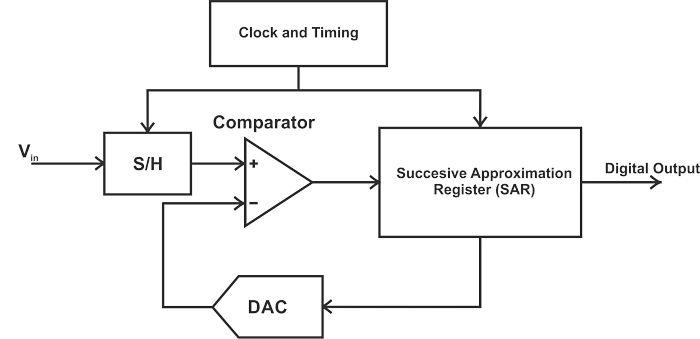##### Figure 3. Block diagram for a SAR ADC.

The first step in the SAR digitization algorithm is the sampling phase, during which the S/H acquires the input value. This sample will be held for the entire conversion phase. During the conversion phase, the acquired sample is successively compared with appropriate threshold levels to find the digital equivalent of the input. To determine every bit of the output, one clock cycle is required. Assuming that the sampling phase also takes one clock cycle, we need an N + 1 clock cycle for an N-bit SAR ADC. Figure 4 shows the S/H output and threshold waveforms for a 3-bit SAR ADC.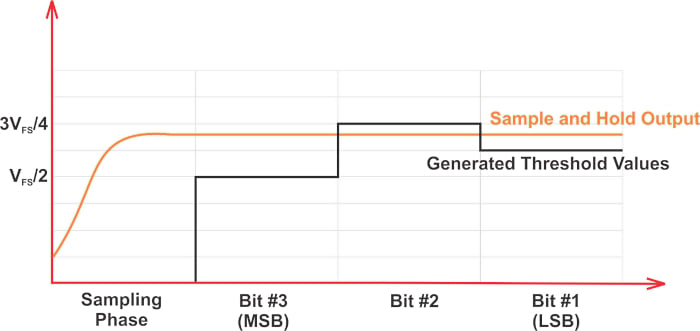##### Figure 4. An S/H output and threshold waveform for a 3-bit SAR ADC.

The important point here is that, for a given conversion phase, the circuit components following the S/H are ideally working with a DC signal no matter what the input frequency is. Therefore, any nonlinearity within the comparator or the internal DAC (digital-to-analog converter) of a SAR ADC is not going to change with the input frequency. We can say that the nonlinearity of the encoder portion of the ADC contributes to the static (or DC) nonlinearity of the system. Static nonlinearity is characterized by DNL (differential nonlinearity) and INL (integral nonlinearity) errors in ADC’s transfer function.

What about the S/H nonlinearity? Unlike the encoder portion that effectively deals with a DC signal, the S/H “sees” an AC signal. We’ll discuss in the next section how a significant portion of the S/H nonlinearity changes with the input frequency. As a result, the S/H determines the dynamic (or AC) linearity of the ADC.

### S/H Circuit Nonlinearity

To have an idea about the S/H nonlinearity, consider the simple S/H circuit shown in Figure 5.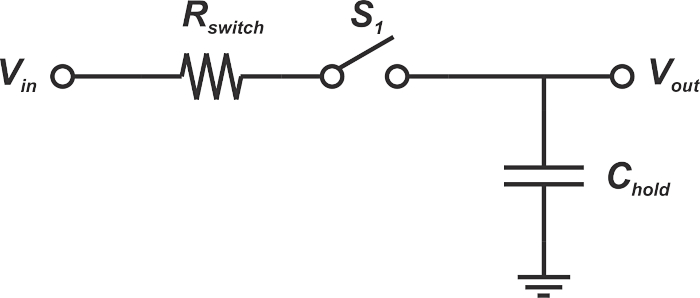##### Figure 5. Example S/H circuit.

This basic S/H consists of a sampling switch, S1, and a hold capacitor, (Chold), which is used to store the acquired sample.

The circuit operation consists of two modes: the sampling (or acquisition mode) and the hold mode. In the sampling mode, the switch is on, and the capacitor voltage tracks the input. At the sampling instant, the switch turns off and disconnects Chold from the input. This starts the hold mode, where the capacitor holds the acquired sample.

In practice, we cannot have an ideal switch with zero resistance. To highlight this, the above diagram explicitly shows the switch resistance, Rswitch. The thermal noise of the switch resistance is a dominant noise contributor in high-resolution Nyquist rate ADCs. To get around this, the value of the hold capacitor is normally chosen to be large enough to limit the bandwidth and, consequently, the noise of the system. However, a limited bandwidth means that the output of the S/H cannot instantaneously reach its final value. This is due to the time constant of the RC network, which is given by $$\tau = R_{switch}C_{hold}$$.

Figure 6 shows example waveforms for one cycle of the S/H operation.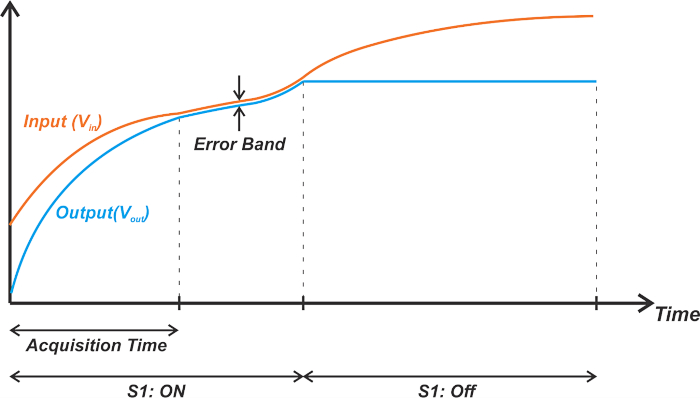##### Figure 6. Example waveforms for one cycle of the S/H circuit operation.

The S/H needs some time—shown by “Acquisition Time” in the figure—to settle within a specified error band around the final value. After the acquisition time, the S/H is able to track the input with a small error. The acquisition time depends on the value of Rswitch, Chold, and the maximum allowable error. Additionally, the acquisition time puts an upper limit on the maximum sampling rate of the ADC.

In practice, the switch resistance is not constant and can change with the input level. The dependence of Rswitch on the input can cause input-dependent phase shift and thus harmonic distortion. Figure 7 shows example waveforms for a case where Rswitch increases with the input level.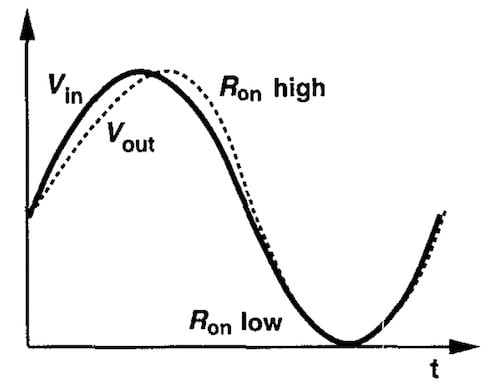##### Figure 7. Example waveforms for when Rswitch increases with the input level. Image used courtesy of B. Razavi

Note that this phase shift (or nonlinearity) changes with frequency. For example, at frequencies much smaller than the pole of the RC network, we have zero phase shift, and the small variations in Rswitch should have a negligible effect on linearity. However, as we increase the frequency, the phase shift becomes more and more significant.

It’s worthwhile to mention that the variation of Rswitch with input is only one source of S/H nonlinearity. Mechanisms such as input-dependent charge injection of the switch, as well as input-dependent sampling instant, are other phenomena that lead to S/H nonlinearity. The latter mechanism refers to the fact that the instant at which the switch turns off can change with the input level.

### Slew Rate Limit Issue

The frequency-dependent nonlinearity of an S/H circuit can also be explained by noting that the circuit driving the hold capacitor has a limited slew rate. Figure 8 shows the block diagram of a typical S/H circuit in greater detail.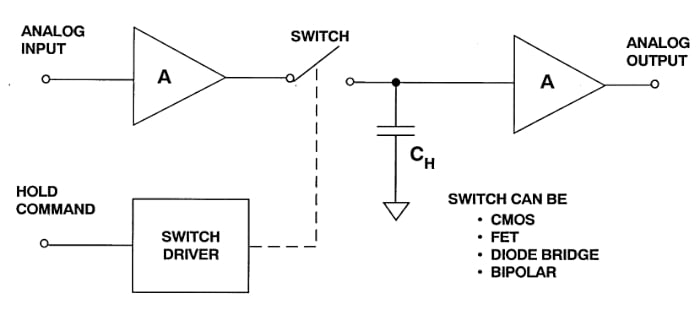##### Figure 8. A more detailed S/H circuit block diagram. Image used courtesy of Analog Devices.

In this circuit, the first amplifier buffers the input by presenting a high impedance to the signal source. It also provides current gain to charge the hold capacitor. The right-hand amplifier acts as an output buffer and prevents the S/H output voltage from being discharged by the input impedance of the following circuit during the hold mode. Assume that the short-circuit output current of the input buffer is ISC. This is the maximum current that the buffer can supply to CH. Therefore, the slew rate (or the maximum rate of change of the S/H output) is given by Equation 1.

$Slew \text{ } Rate = \frac{\Delta V}{\Delta t}=\frac{I_{SC}}{C_{H}}$

##### Equation 1.

For a sine wave input:

$V_{in}=V_M sin(2 \pi ft)$

The maximum rate of change of the signal is given by:

$max \big(\frac{dV_{in}}{dt}\big)=2 \pi fV_M$

For a given large signal input, increasing the frequency can make the rate of change of the signal greater than the slew rate of the S/H. In this case, the S/H output is not able to follow the input quickly enough, leading to signal distortion problems. The lack of S/H that exhibits an adequate slew rate to keep up with the rapidly changing analog input is a key reason why many ADCs fail to perform well beyond several megahertz of signal bandwidth.

As an example, consider the AD9042 from Analog Devices. Although the AD9042 is a converter specifically designed with a wideband, high SFDR front end, its SFDR still degrades with the input frequency, as shown in Figure 9.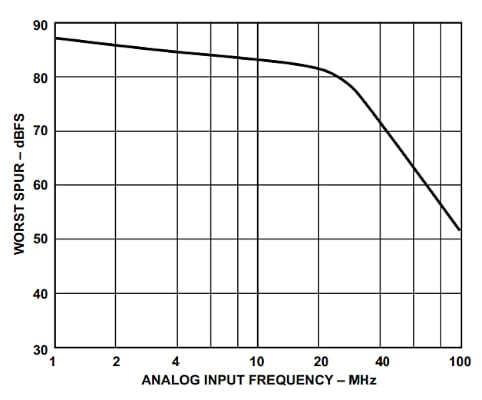##### Figure 9. Graph showing how theAD9042's SFDR degrades with the input frequency. Image used courtesy of Analog Devices.

The above discussion also explains the SNR-SFDR trade-off we mentioned earlier in this article. Note that a larger hold capacitor leads to a lower slew rate (Equation 1) and higher distortion (or lower SFDR). On the other hand, a larger capacitor reduces the system bandwidth and improves the noise performance (higher SNR).

### Applying Dithering to Improve SFDR

As discussed above, there are two main limitations to improving the SFDR: the nonlinearity produced by the S/H circuit and that from the encoder portion of the ADC. There is nothing that can be done externally to reduce the distortion produced by the S/H circuit. However, the dithering technique can reduce the nonlinearity from the encoder portion of the ADC. This will be discussed in the next article in this series.